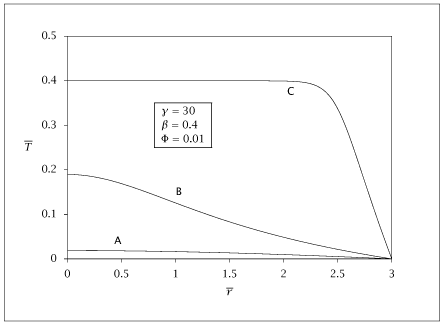## Figure 7.21:

### Dimensionless temperature versus radius for the nonisothermal spherical pellet: lower (A), unstable middle (B), and upper (C) steady states.## Code for Figure 7.21

### main.m

```
1
2
3
4
5
6
7
8
9
10
11
12
13
14
15
16
17
18
19
20
21
22
23
24
25
26
27
28
29
30
31
32
33
34
35
36
37
38
39
40
41
42
43
44
45
46
47
48
49
50
51
52
53
54
55
56
57
58
59
60
61
62
63
64
65
66
67
68
69
70
71
72
73
74
75
76
77
78
79
80
81
82
83
84
85
86
87
88
89
90
91
92
93
94
95
96
97
98
99
100
101
102
103
104
105
106
107
108
109
110
111
112
113
114
115
116
117% Copyright (C) 2001, James B. Rawlings and John G. Ekerdt
%
% This program is free software; you can redistribute it and/or
% modify it under the terms of the GNU General Public License as
% published by the Free Software Foundation; either version 2, or (at
% your option) any later version.
%
% This program is distributed in the hope that it will be useful, but
% WITHOUT ANY WARRANTY; without even the implied warranty of
% MERCHANTABILITY or FITNESS FOR A PARTICULAR PURPOSE.  See the GNU
% General Public License for more details.
%
% You should have received a copy of the GNU General Public License
% along with this program; see the file COPYING.  If not, write to
% the Free Software Foundation, 59 Temple Place - Suite 330, Boston,
% MA 02111-1307, USA.

%
% compute the pellet temperature and concentration profiles
% for the multiple steady state Weisz Hicks problem
%
% 7/18/01
%
% jbr
%
% Revised 8/14/2018

Gamma = 30;
beta  = 0.4;
Phiscale  = 0.01;
tol = 1e-6;

if (exist ('OCTAVE_VERSION')) % IF OCTAVE
[value, ier, nfun, err] = quad(@(c) integrand(c, beta, Gamma), 0, 1, tol);

if (ier ~= 0)
fprintf ('darn, quad failed on integral\n nfun and err =\n');
nfun, err
end

else % ELSE
[value, nfun] = quadl(@(c) integrand(c, beta, Gamma), 0, 1, tol);
end % ENDIF

intercept = sqrt(2*value);
Phi = intercept*Phiscale;

rout = linspace(0,3,100)';
%c0=1e-11;
c0vec = [1e-11; 0.5; 0.95];
nc = length(c0vec);
results(1:nc) = {[]};

for i = 1:length(c0vec)
c0 = c0vec(i);
x0 = [c0; 0; 1; 0];
ode_opts = odeset();
rel = ode_opts.RelTol;

if (isempty (rel))
rel = sqrt (eps);
end

ode_opts = odeset('AbsTol', rel*c0, 'RelTol', sqrt(eps));
[rsolver,xout] = ode15s(@(r, x) pelletode(r, x, beta, Gamma, Phi),...
rout, x0, ode_opts);
%
% checked sensitivies by finite difference, done 7/18/01
%
ceinit = xout(length(rout),1);
c0init = c0;
y0     = [ceinit; c0init];
ydot0  = [-1; -1/xout(length(rout), 3)];
tsteps = linspace(0, ceinit - 1, 10)';
dae_opts = odeset ('AbsTol', rel*c0init, 'RelTol', sqrt(eps));
[tsolver,y]  = ode15i(@(t, y, ydot) continode(t, y, ydot, beta, Gamma, Phi, rout),...
tsteps, y0, ydot0, dae_opts);
%
% solve the pellet problem from correct c0
%
c0 = y(length(tsteps),2);
x0 = [c0; 0; 1; 0];

ode_opts = odeset('AbsTol', rel*c0, 'Reltol', sqrt(eps));
[rsolver,xout] = ode15s(@(r, x) pelletode(r, x, beta, Gamma, Phi),...
rout, x0, ode_opts);

conc = xout(:,1);
temp = beta*(1 - conc);
eta(i) = xout(length(rout),2)/Phi^2;
results{i} = [rsolver, conc, temp];
end

save whmss.dat results;

if (~ strcmp (getenv ('OMIT_PLOTS'), 'true')) % PLOTTING
subplot (2, 1, 1);
hold on

for i = 1:nc
plot (results{i}(:,1), results{i}(:,2));
end

% TITLE whmss
subplot (2, 1, 2);
hold on

for i = 1:nc
plot (results{i}(:,1), results{i}(:,3));
end

% TITLE whmssT
hold off
end % PLOTTING

```

### integrand.m

```
1
2function retval = integrand(c, beta, Gamma)
retval = c *exp(Gamma*beta*(1-c)/(1+beta*(1-c)));

```

### pelletode.m

```
1
2
3
4
5
6
7
8
9
10
11
12
13
14
15
16function xdot = pelletode(r, x, beta, Gamma, Phi)
ca    = x(1);
s1    = x(3);
s2    = x(4);
xdot = zeros (4, 1);
if (r == 0)
xdot(2) = 1/3* Phi^2*ca*exp(Gamma*beta*(1-ca)/(1+beta*(1-ca)));
xdot(4) = 0;
else
tmp = Phi^2*ca*exp(Gamma*beta*(1-ca)/(1+beta*(1-ca)));
xdot(2) = - 2/r*dcadr + tmp;
xdot(4) = tmp*(1/ca - Gamma*beta/(1+beta*(1-ca))^2)*s1 - 2/r*s2;
end
xdot(3) = s2;

```

### continode.m

```
1
2
3
4
5
6
7
8
9
10
11
12
13
14function res = continode(t, y, ydot, beta, Gamma, Phi, rout)
ce = y(1);
c0 = y(2);
%
% solve pellet problem from c0
%
x0=[c0; 0; 1; 0];
ode_opts = odeset('AbsTol', 1e-5*c0, 'RelTol', sqrt(eps));
[rsolver,xout] = ode15s(@(r, x) pelletode(r, x, beta, Gamma, Phi),...
rout, x0, ode_opts);
%
res = zeros (2, 1);
res(1) = ydot(1) + 1;
res(2) = ydot(2) + 1/xout(length(rout),3);

```